• 非线性优化的基本概念 定义：如果目标函数或约束条件中至少有一个是非线性函数时的最优化问题就叫做非线性规划问题。 一般形式： min f ( X ) gi( X ) ≥ 0 , i = 1,2,…,m; hj( X ) ≥ 0 , j = 1,2,…,m; 其中 X = ...
非线性优化的基本概念
定义：如果目标函数或约束条件中至少有一个是非线性函数时的最优化问题就叫做非线性规划问题。
一般形式：
min f ( X )
gi( X ) ≥ 0 ,       i = 1,2,…,m;
hj( X ) ≥ 0 ,       j = 1,2,…,m;
其中 X = ( X1 , X2 ,…, Xn )T ∈ En ，f ，gi，hj 是定义在 En 上的实值函数。
其他情况：求目标函数的最大值或约束条件为小于等于零的情况，都可通过取其相反数化为上述一般形式。
解的定义：

把满足问题一般形式中条件的解 X 称为可行解（或可行点），所有可行点的集合称为可行集（或可行域）记为 D ，即 D = { X | gi( X ) ≥ 0 ,  hj( X ) ≥ 0 , X ∈ En }
设 X* ∈ D ，若存在 σ＞0 ，使得对一切  X∈ D 且 || X -  X* ||＜σ，都有  f ( X* ) ＜ f ( X )，则 X* 是 f ( X ) 在D上的局部极小值点（局部最优解）。特别地当 X ≠ X* 时，若  f ( X* ) ＜ f ( X ) ，则称X*是 f ( X ) 在D上的严格局部极小值点（严格局部最优解）。
设 X* ∈ D ，对任意的 X ∈ D ,都有  f ( X* ) ＜ f ( X ) ，则称X是f(X)在D上的全局极小值点（全局最优解）。特别地当 X ≠ X* 时，若  f ( X* ) ＜ f ( X ) ，则称X是 f ( X ) 在D上的严格全局极小值点（严格全局最优解）。

非线性优化的基本解法
罚函数法
罚函数法基本思想是通过构造罚函数把约束问题转化为一系列无约束最优化问题，进而用无约束最优化方法去求解．这类方法称为序列无约束最小化方法．简称为SUMT法．

SUMT外点法

对于一般的非线性规划，可设：T ( X , M ) = f ( X ) + M ∑ [ min ( 0 , gi ( X ) ) ]2 + M ∑ [ hj ( X ) ]2，即将非线性优化问题转化为无约束问题 min T ( X , M )。
其中 T ( X , M ) 称为罚函数，M 称为罚因子，带 M 的项称为罚项，这里的罚函数只对不满足约束条件的点实行惩罚：当 X ∈ D 时，满足各 gi( X ) ≥ 0 ,  hj( X ) ≥ 0 ，故罚项 = 0，不受惩罚。当 X ∉ D 时，必有                 的约束条件，故罚项 > 0，要受惩罚。

罚函数法的缺点是：每个近似最优解 Xk 往往不是容许解，而只能近似满足约束，在实际问题中这种结果可能不能使用；在解一系列无约束问题中，计算量太大，特别是随着 Mk 的增大，可能导致错误．

SUMT内点法

设集合D0 = { X | gi ( X ) >0 , i = 1,2,…,m } ≠ 空集，D0 是可行域内所有严格内点的集合。
构造障碍函数 I ( X , r ) = f ( X ) + r∑ lngi ( X ) ，其中 r∑ lngi ( X ) 为障碍项，r 为障碍因子，这样问题就转化成一系列求极值问题。
近似规划法
近似规划法的基本思想：将目标函数和约束条件近似为线性函数，并对变量的取值范围加以限制，从而得到一个近似线性规划问题，再用单纯形法求解之，把其符合原始条件的最优解作为解的近似．每得到一个近似解后，都从这点出发，重复以上步骤，这样，通过求解一系列线性规划问题，产生一个由线性规划最优解组成的序列，经验表明，这样的序列往往收敛于非线性规划问题的解。
算法步骤：

给定初始可行点 X1 = { x11，x21,…,xn1}，步长限制 σj1 ( j = 1,…,n )，步长缩小系数 β ∈ ( 0 , 1 )，允许误差 ε ，令k=1；

在点 Xk 处，将 f ( X )，gi( X )，hj( X ) 按泰勒级数展开并取一阶近似，得到近似线性规划问题：
min  f ( X ) ≈ f ( Xk ) + ▽ f ( Xk )T ( X - Xk )
gi( X ) ≈ gi ( Xk ) + ▽ gi ( Xk )T ( X - Xk ) ≥ 0
hj( X ) ≈ hj ( Xk ) + ▽ hj ( Xk )T ( X - Xk ) = 0

在上述近似线性规划问题的基础上增加一组限制步长的线性约束条件。因为线性近似通常只在展开点附近近似程度较高，故需要对变量的取值范围加以限制，所增加的约束条件是：| xj - xjk | ≤ σjk
求解该线性规划问题，得到最优解 ；

检验 Xk+1 点对原约束是否可行。若对原约束可行，则转步骤5；否则，缩小步长限制，令 σjk =  β σjk  ，返回步骤3，重解当前的线性规划问题。

判断精度：若 | σjk | ＜ ε，则点 Xk+1 为近似最优解；否则，令  σjk+1 = σjk ，k=k+1，返回步骤2。

MATLAB解非线性优化问题
二次规划
标准型为：
min Z = 1/2XTHX + CT X
AX ≤ b，Aeq * X = beq，VLB ≤ X ≤VUB
常用格式如下：
   1.	x = quadprog ( H, C, A, b);
2.	x = quadprog ( H, C, A, b, Aeq, beq);
3.	x = quadprog ( H, C, A, b, Aeq, beq, VLB, VUB);
4.	x = quadprog ( H, C, A, b, Aeq, beq, VLB, VUB, X0);
5.	x = quadprog ( H, C, A, b, Aeq, beq, VLB, VUB, X0, options);
6.	[x, fval] = quadprog (...) ;
7.	[x, fval, exitflag] = quadprog (...);
8.	[x, fval, exitflag, output] = quadprog (...);

一般非线性规划
标准型为：
min F ( X )
AX ≤ b，Aeq * X = beq，G (X) ≤ 0，Ceq ( X ）= 0，VLB ≤ X ≤VUB
首先建立M文件fun.m，定义目标函数 F (X) ，若约束条件中有非线性约束:G(X) 或 Ceq ( X ) = 0，则建立M文件nonlcon.m定义函数 G  (X ) 与 Ceq ( X )。
常用格式如下：
   1.  x = fmincon ( ‘fun’, X0, A, b)
2.  x = fmincon ( ‘fun’, X0, A, b, Aeq, beq)
3.  x = fmincon ( ‘fun’, X0, A, b, Aeq, beq, VLB, VUB)
4.  x = fmincon ( ‘fun’, X0, A, b, Aeq, beq, VLB, VUB, ’nonlcon’)
5.  x = fmincon ( ‘fun’, X0, A, b, Aeq, beq, VLB, VUB, ’nonlcon’, options)
6.  [x, fval] = fmincon(...)
7.  [x, fval, exitflag] = fmincon(...)
8.  [x, fval, exitflag, output] = fmincon(...)


fmincon函数的中型算法使用的是序列二次规划法。在每一步迭代中求解二次规划子问题，并用BFGS法更新拉格朗日Hessian矩阵。
fmincon函数可能会给出局部最优解，这与初值X0的选取有关。


展开全文matlab 数学建模
• 非线性规划模型2.01规划模型五、LINGO代码1.非线性规划模型2.01规划模型六、实际案例七、论文案例片段（待完善） 线性规划模型主要针对数学建模问题中的一些小的子问题进行求解，如果想直接使用请跳转至——四、五 ...
文章目录一、算法介绍1. 01规划模型二、适用问题三、算法总结四、应用场景举例1.非线性规划模型2.01规划模型五、LINGO代码1.非线性规划模型2.01规划模型六、实际案例七、论文案例片段（待完善）

非线性规划模型与01规划模型模型主要针对数学建模问题中的一些小的子问题进行求解，如果想直接使用请跳转至——四、五
视频回顾

一、算法介绍
1. 01规划模型
01规划是指未知量的取值范围只能是0,1的规划问题，
通常是线性规划
二、适用问题
三、算法总结
四、应用场景举例
1.非线性规划模型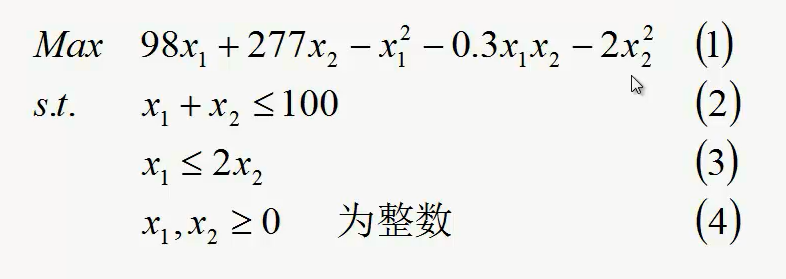2.01规划模型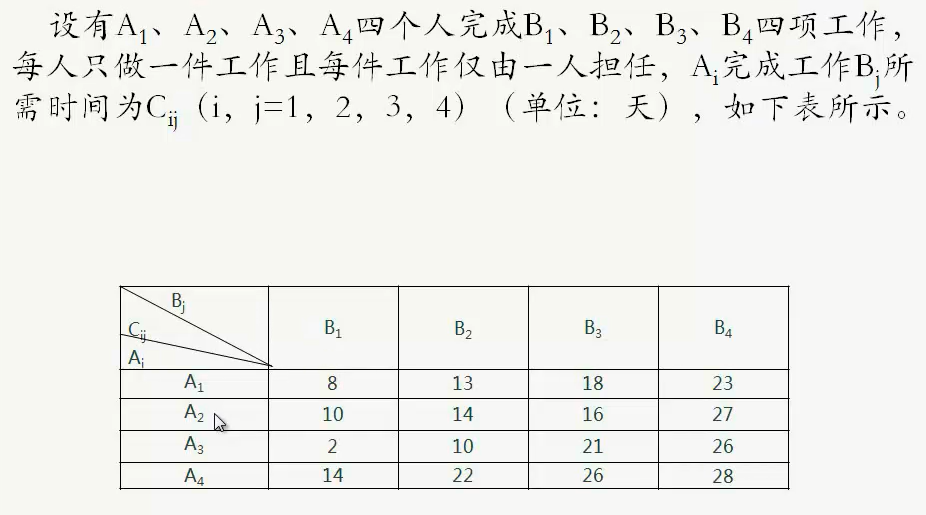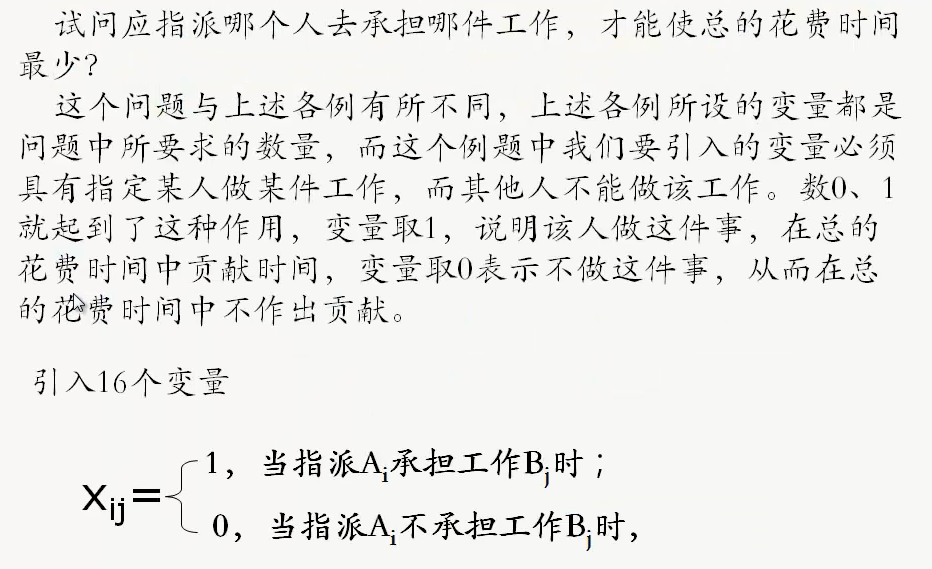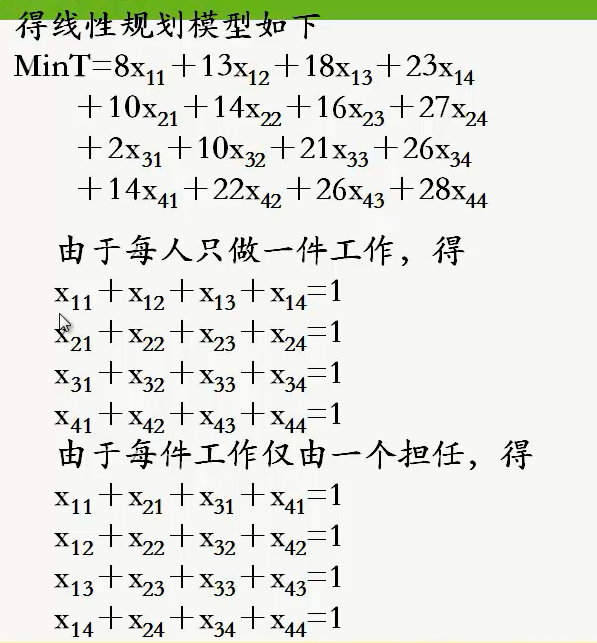五、LINGO代码
1.非线性规划模型
Model:
max=98*x1+277*x2-x1*x1-0.3*x1*x2-2*x2*x2;
x1+x2<100;
x1<=2*x2;
@gin(x1);
@gin(x2);
end

2.01规划模型
Model:
Min=8*x11+13*x12+18*x13+23*x14+10*x21+14*x22+16*x23+27*x24+2*x31+10*x32+21*x33+26*x34+14*x41+22*x42+26*x43+28*x44;
x11+x12+x13+x14=1;
x21+x22+x23+x24=1;
x31+x32+x33+x34=1;
x41+x42+x43+x44=1;
x11+x21+x31+x41=1;
x12+x22+x32+x42=1;
x13+x23+x33+x43=1;
x14+x24+x34+x44=1;
end
int16


六、实际案例


七、论文案例片段（待完善）


展开全文算法 机器学习 数学建模 人工智能
• 注：该博客是在学习过程中 对知识点的整理 以方便日后查阅 照片丢掉的是深度信息 小孔成像原理 α是在u轴缩放 α倍， β是在v轴上缩放β倍 单位为 像素/米 ...双目相机模型原理： （根据三角形相似得出） ...
注：该博客是在学习过程中 对知识点的整理 以方便日后查阅
照片丢掉的是深度信息
小孔成像原理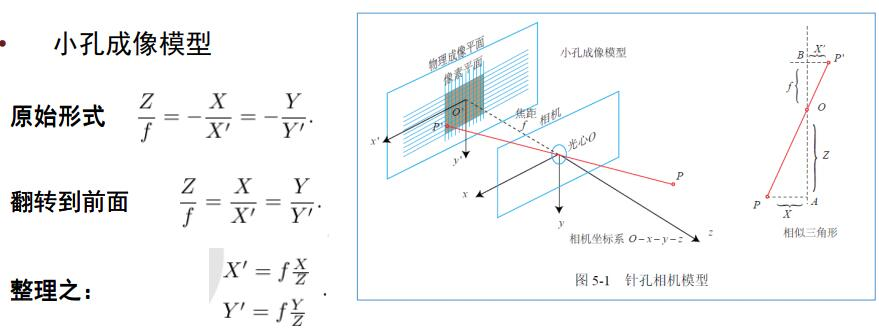α是在u轴缩放 α倍， β是在v轴上缩放β倍 单位为 像素/米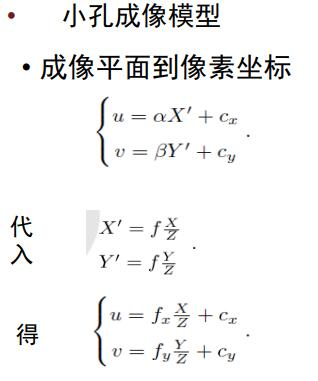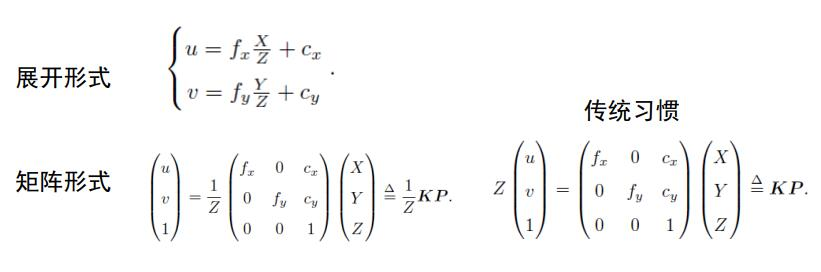归一化平面是说 当 向量（X,Y,Z）T 向量缩放或扩大多少倍后 在p点在相机的投影 是同一点
（同一直线上的投影点是一样的）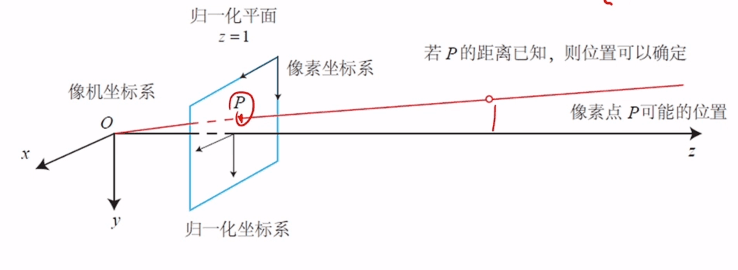相机坐标系和世界坐标系的变换：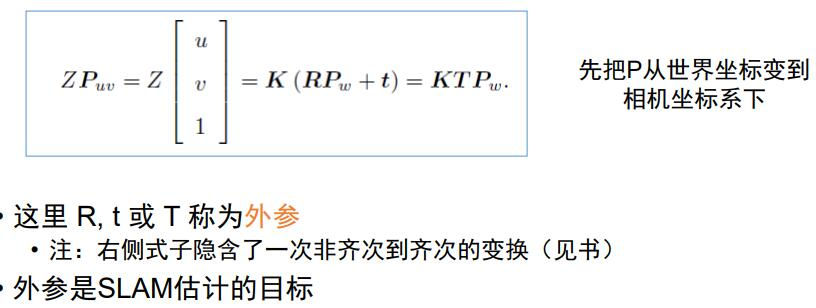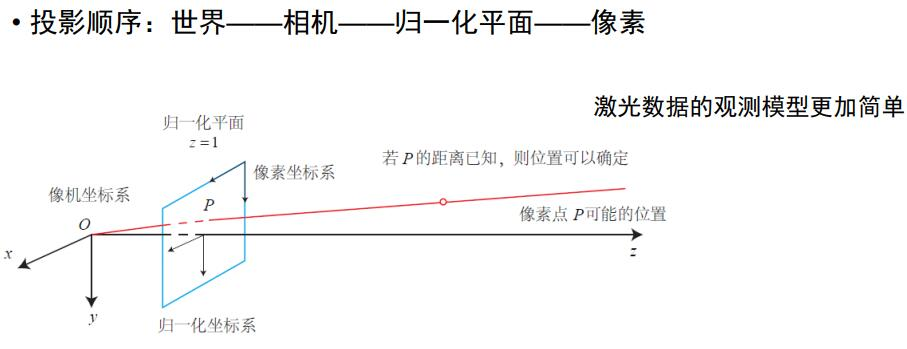双目相机模型原理： （根据三角形相似得出）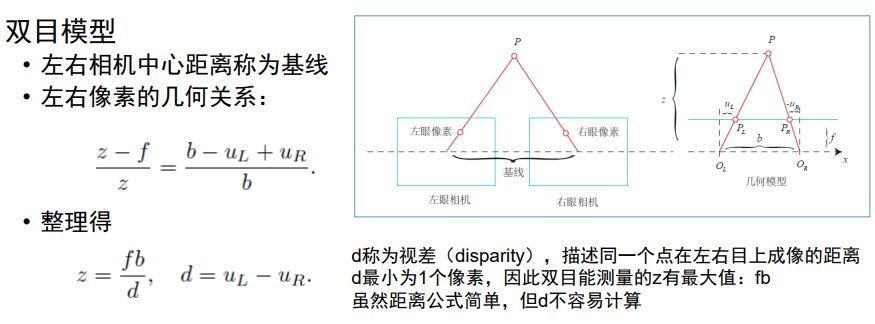RGB-D相机：
结构光 或 飞行时间原理（激光原理）

图像
灰度图（8位整数）
深度图（16位整数）
彩色图（多通道）

批量状态估计问题（batch）
给多 时刻的 u z 推出 x，y  :批量
已知t=k 时刻的x，y 求出 t= k+1 时刻的x， y ：递归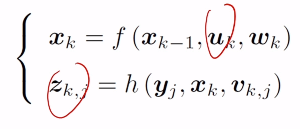批量式（batch）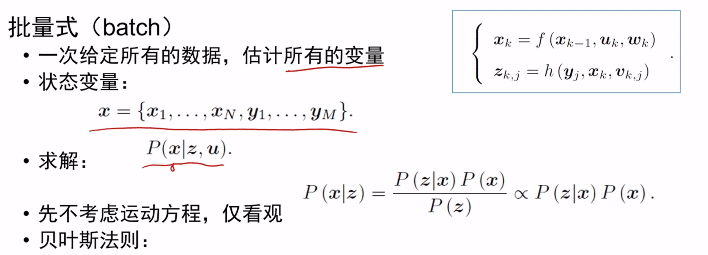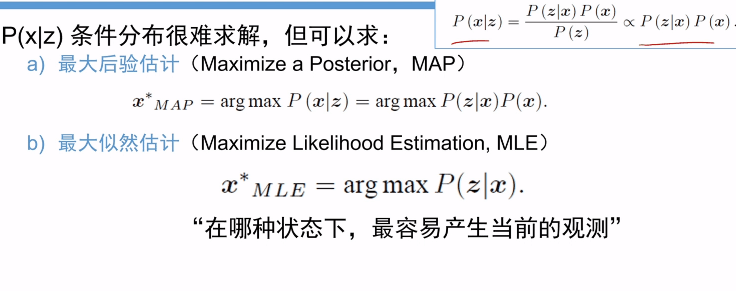举例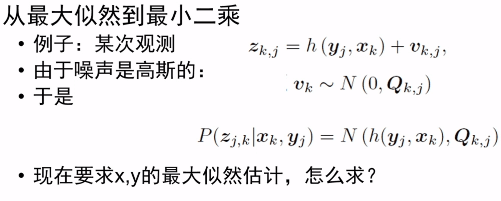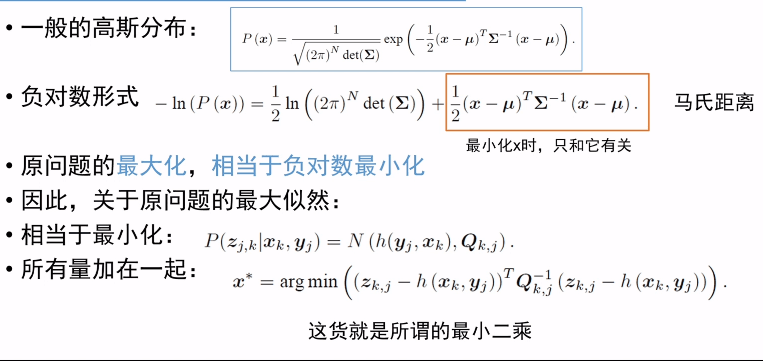问题转换：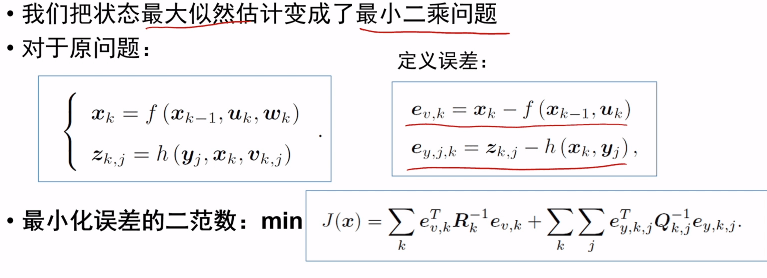非线性优化（最小二乘问题）
简单问题：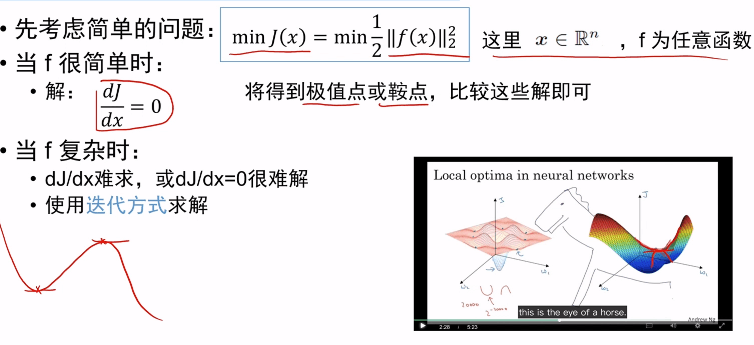迭代方式：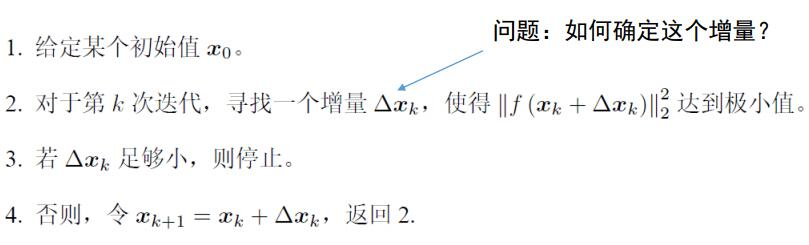确定增量（梯度下降策略）： 一阶或二阶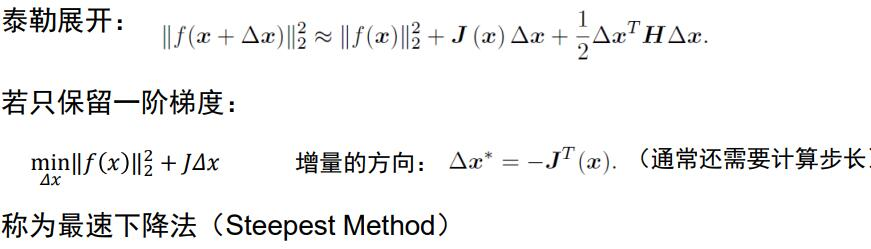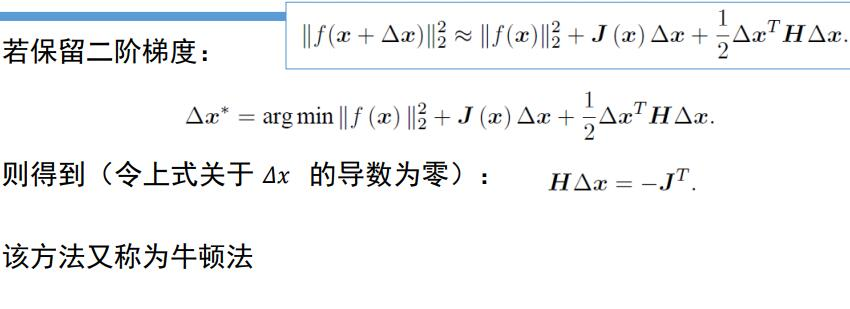最速下降发挥碰到zigzag问题(过于贪婪 导致走直角线）
牛顿法迭代次数少，但是Hessian矩阵计算过于复杂

为避免Hessian 矩阵计算 可用方法有：
1。Gauss-Newton
用二阶近似，但是回避Hessian计算：
但H逆可能不存在
2。Levenberg-Marquadt （改善1。方法）
展开全文• ## G2O非线性优化

千次阅读 2017-02-16 13:07:45
G2O非线性优化 上一篇文章介绍最小二乘法，本文介绍g2o实现最小二乘法。 g2o即General Graphic Optimization，它是一个基于图优化的库。至于图优化理论，参照半闲居士的博客 接着上回曲线拟合问题，拟合y=Asin(Bx...
上一篇文章介绍最小二乘法，本文介绍g2o实现最小二乘法。
g2o即General Graph Optimization，它是一个基于图优化的库。至于图优化理论，参照半闲居士的博客
接着上回曲线拟合问题，拟合y=Asin(Bx)+Ccos(Dx)<!--//--><![CDATA[//><!--
y=Asin(Bx)+Ccos(Dx)
//--><!]]>，已知N组数据(xi,yi),i=0,1,⋯N−1<!--//--><![CDATA[//><!--
(x_i, y_i), i = 0, 1, \cdots N-1
//--><!]]>，待优化变量V=[A,B,C,D]<!--//--><![CDATA[//><!--
V = [A, B, C, D]
//--><!]]>，优化问题即为： V=argmin(∑i=0N−1(y−Asin(BX)−Ccos(Dx))2)<!--//--><![CDATA[//><!--
V = {argmin} \left(\sum_{i=0}^{N-1}(y-Asin(BX)-Ccos(Dx))^2 \right)
//--><!]]>
整个图中只有一个顶点，即待优化变量V，连接只有一个顶点的边即一元边（Unary Edge），使用g2o主要有以下几个步骤：
1. 定义顶点和边的类型；
2. 选择优化算法；
3. 构建图；
4. 调用 g2o 进行优化，返回结果。

由于g2o中没有本例类型顶点和边，因此首先需要自己定义。节点和边定义的方式，都是继承BaseVertex和BaseEdge，可以参考优化相机位姿和3D点坐标常用几个类型VertexSE3Expmap、VertexSBAPointXYZ、EdgeSE3ProjectXYZ、EdgeSE3ProjectXYZOnlyPose定义代码，熟悉这种这种模板定义的方式。

// 曲线模型的顶点(要优化的元素)
// vertex dimension: 4
// vertex type: Vector4d
class CurveFittingVertex: public g2o::BaseVertex<4, Vector4d>
{
public:
EIGEN_MAKE_ALIGNED_OPERATOR_NEW

CurveFittingVertex();

virtual void setToOriginImpl()  // 重置
{
_estimate << 0, 0, 0, 0;
}

virtual void oplusImpl(const double* update_);// 更新

//输入输出可以不定义
bool write(std::ostream& os) const {}
};

// 顶点构造函数
CurveFittingVertex::CurveFittingVertex() : BaseVertex<4, Eigen::Vector4d>()
{
}

// 顶点更新函数
void CurveFittingVertex::oplusImpl(const double* update_)
{
Eigen::Map<const Vector4d> up(update_);
_estimate += up;
}

顶点的定义主要需要定义_estimate的更新函数oplusImpl和setToOriginImpl。

// 曲线模型的边(要优化的误差)
// unary edge to optimize error
// error vector dimensition: 1
// measurement type: double
// Vertex type: CurveFittingVertex
class CurveFittingEdge: public g2o::BaseUnaryEdge<1, double, CurveFittingVertex>
{
public:
EIGEN_MAKE_ALIGNED_OPERATOR_NEW
CurveFittingEdge();

// 计算曲线模型误差
void computeError();

virtual void linearizeOplus();

bool write(std::ostream& os) const {}

public:
double _x;
};

// 边的构造函数
CurveFittingEdge::CurveFittingEdge() : g2o::BaseUnaryEdge<1, double, CurveFittingVertex>()
{

}
// 误差函数
void CurveFittingEdge::computeError()
{
const CurveFittingVertex* v = static_cast<const CurveFittingVertex*>( _vertices);
const Vector4d abcd = v->estimate();
double A = abcd, B = abcd, C = abcd, D = abcd;
_error(0,0) = _measurement - (A * sin(B*_x) + C * cos(D*_x));  // 误差函数：观测量减去估计量
}

// Jacobin
void CurveFittingEdge::linearizeOplus()
{
CurveFittingVertex *vi = static_cast<CurveFittingVertex *>(_vertices);
Vector4d abcd = vi->estimate();
double A = abcd, B = abcd, C = abcd, D = abcd;
// 误差项对待优化变量的Jacobin
_jacobianOplusXi(0,0) = -sin(B*_x);
_jacobianOplusXi(0,1) = -A*_x*cos(B*_x);
_jacobianOplusXi(0,2) = -cos(D*_x);
_jacobianOplusXi(0,3) = C*_x*sin(D*_x);
}

误差函数e=y−Asin(Bx)−Ccos(Dx)<!--//--><![CDATA[//><!--
e = y - Asin(Bx)-Ccos(Dx)
//--><!]]>,因此 ∂e∂[ABCD]=[−sin(Bx)  −Axcos(Bx)  −cos(Dx)  Cxsin(Dx)]<!--//--><![CDATA[//><!--
\frac{\partial e}{\partial [ABCD]} = [-sin(Bx) \ \ -Axcos(Bx) \ \ -cos(Dx) \ \ Cxsin(Dx)]
//--><!]]>
边的定义主要需要定义误差函数和计算Jacobin。
当然边的Jacobin函数可以不定义，g2o会采用如上篇博客的数值求导方法。如果我们能够推导出雅可比矩阵的解析形式并告诉优化库，就可以避免数值求导中的诸多问题。

然后是图的构造和求解了

#include <iostream>
#include <g2o/core/base_vertex.h>
#include <g2o/core/base_unary_edge.h>
#include <g2o/core/block_solver.h>
#include <g2o/core/optimization_algorithm_levenberg.h>
#include <g2o/core/optimization_algorithm_gauss_newton.h>
#include <g2o/core/optimization_algorithm_dogleg.h>
#include <g2o/solvers/dense/linear_solver_dense.h>

#include <Eigen/Core>
#include <Eigen/Dense>

#include <opencv2/core/core.hpp>
#include <cmath>

#include <chrono>

using namespace std;
using namespace Eigen;
int main()
{
double a = 5.0, b = 1.0, c = 10.0, d = 2.0; // 真实参数值
int N = 100;
double w_sigma = 2.0;   // 噪声值Sigma
cv::RNG rng;    // 随机数产生器OpenCV
double abcd = {0, 0, 0, 0};  // 参数的估计值abc

vector<double> x_data, y_data;

cout << "generate random data" << endl;

for(int i = 0; i < N; i++)
{
//generate a random variable [-10 10]
double x = rng.uniform(-10., 10.);
double y = a * sin(b*x) + c * cos(d *x) + rng.gaussian(w_sigma);
x_data.push_back(x);
y_data.push_back(y);

cout << x_data[i] << " , " << y_data[i] << endl;
}

// 构建图优化，先设定g2o
// 矩阵块：每个误差项优化变量维度为4 ，误差值维度为1
typedef g2o::BlockSolver< g2o::BlockSolverTraits<4, 1> > Block;
// 线性方程求解器：稠密的增量方程
Block::LinearSolverType* linearSolver = new g2o::LinearSolverDense<Block::PoseMatrixType>();

Block* solver_ptr = new Block(linearSolver);    // 矩阵块求解器

// 梯度下降方法，从GN, LM, DogLeg 中选
g2o::OptimizationAlgorithmLevenberg* solver = new g2o::OptimizationAlgorithmLevenberg( solver_ptr );
// g2o::OptimizationAlgorithmGaussNewton* solver = new g2o::OptimizationAlgorithmGaussNewton( solver_ptr );
// g2o::OptimizationAlgorithmDogleg* solver = new g2o::OptimizationAlgorithmDogleg( solver_ptr );

g2o::SparseOptimizer optimizer;     // 图模型
optimizer.setAlgorithm( solver );   // 设置求解器
optimizer.setVerbose(true);     // 打开调试输出

// 往图中增加顶点
CurveFittingVertex *v = new CurveFittingVertex();
// 设置优化初始估计值
v->setEstimate( Eigen::Vector4d(1.6, 1.4, 6.2, 1.7));
v->setId(0);
v->setFixed(false);

// 往图中增加边
for(int i = 0; i < N; i++)
{
CurveFittingEdge* edge = new CurveFittingEdge();
edge->setId(i+1);
edge->setVertex(0, v);      // 设置连接的顶点
edge->setMeasurement( y_data[i] );      // 观测数值

// 信息矩阵：协方差矩阵之逆
edge->setInformation( Eigen::Matrix<double, 1, 1>::Identity() * 1 /(w_sigma* w_sigma) );

edge->_x = x_data[i];

}

// 执行优化
cout << "strat optimization" << endl;

optimizer.initializeOptimization();
optimizer.optimize(100);

chrono::duration<double> time_used = chrono::duration_cast<chrono::duration<double> > (t2 - t1);
cout << "solve time cost = " << time_used.count() << " seconds." << endl;

// 输出优化值
Eigen::Vector4d abcd_estimate = v->estimate();
cout << "estimated module: " <<  endl << abcd_estimate << endl;

return 0;
}

另外g2o中设置核函数、边缘化等等后续再更新。

引用:
 《g2o: A General Framework for Graph Optimization》
 《slambook by gaoxiang》
展开全文最小二乘法 图优化 x
• 非线性规划问题，是指目标函数或约束函数中至少有一个是决策变量的非线性函数的优化问题。 1.一般数学模型  非线性规划的一般数学模型可表示为    其中，是n维向量，都是的的映射。梯度
• 从线性到非线性模型 1、线性回归，岭回归，Lasso回归，局部加权线性回归 2、logistic回归，softmax回归，最大熵模型 3、广义线性模型 4、Fisher线性判别和线性感知机 5、三层...
• 支持向量机是机器学习领域里最强的几种分类器之一，被广泛的运用于各种分类回归问题，如果不...其基本原理相当简单，但是模型的求解和优化却十分复杂，很难描述清楚，这里我会一步一步，尽我所能分章节的将它总结完善支持向量机 核函数 拉格朗日对偶
• 线性模型 LinearRegression线性回归 Lasso回归：L1正则化有助于生成一个稀疏权值矩阵，进而可以用于特征选择。由此发现power与userd_time特征非常重要。 ...Ridge回归：L2正则化在拟合过程中通常都倾向...非线性模...算法 python 神经网络 机器学习 人工智能
• Hammerstein非线性模型的基于PSO的参数辨识系统的本质就是将参数的辨识问题转换为参数空间优化问题，对整个参数域进行搜索并最终获得最优的参数估计。 本课题，我们需要的参数辨识模型具体描述如下所示： ...
• #include <ceres/ceres.h>//最小二成非线性优化 #include <chrono>//计时 #include <fstream>//文件流 using namespace std; //  代价函数的计算模型 // １．定义一个Functor(拟函数/函数对象)类，其中定义的是...
• 目标函数或约束条件中包含非线性函数 如果线性规划的最优解存在，其最优解只能在其可行域的边界上达到（特别是可行域的顶点上达到）；而非线性规划的最优解（如果最优解存在）则可能在其可行域的任意一点达到。...
• 基本思想是通过构造罚函数把约束问题转化为一系列无约束最优化问题，进而用无约束最优化方法去求解。这类方法为序列无约束最小化方法。简称为SUMT。分为内点法和外点法两种。 罚函数法：将约束条件放入目标函数 （1...数学建模
• 与线性规划不同的是，非线性规划要求目标函数或约束条件中含有非线性函数。相应的求解这类问题就要用到非线性规划的方法。约束条件或者目标函数的放宽使得规划模型更...
• min w= min a*x1+b*x2+c*x3 s.t.x1>1 x2>10 x2为常数，需通过判断x1的条件语句，然后基于相关公式求得 x3为常数，需通过判断x1的条件语句，然后基于相关公式求得 这个应该怎么编写程序呢？
• （i）首先编写M文件mente.m定义目标函数f 和约束向量函数g，程序如下： function [f,g]=mengte(x); f=x(1)^2+x(2)^2+3*x(3)^2+4*x(4)^2+2*x(5)-8*x(1)-2*x(2)-3*x(3)... -x(4)-2*x(5); g(1)=sum(x)-400;...
•matlab
• 我们每个人都会在我们的生活或者工作中遇到各种各样的最...随着学习的深入，博主越来越发现最优化方法的重要性，学习和工作中遇到的大多问题都可以建模成一种最优化模型进行求解，比如我们现在学习的机器学习算法...
• 神经网络中提到的线性模型非线性模型之间的区别就体现在字面意思上：线性模型即仅通过对输入进行线性变换得到输出的网络模型，我们知道在线性代数中矩阵的相乘就是一种线性变换，根据矩阵乘法的结合律可知：一个...
• 线性模型应用很广泛，但在某些场合也有不足。本章举了三个优化的例子。 第一，要求输出是在一个限定范围的可能值中是有原因的。例如，如果要预测一个概率，那么输出就需要介于0和1之间。把一个线性组合用logistc...
• 什么是非线性优化 对于最优化问题，如果目标函数是非线性的，那就是非线性优化问题，如果目标函数是凸函数，那就是凸优化问题。 首先需要说明的是，最初的问题其实是一个最优化问题。所谓的最优化问题其实就是...
• 一元 例题： 代码： 二元 例题： 代码：python
• 非线性优化整理-2.高斯-牛顿法 ） 高斯牛顿法的迭代公式为 x n + 1 = x n − [ J T f J f ] − 1 J T f f ( x n ) x n + 1 = x n − [ J f T J f ] − 1 J f T f ( x n ) x_{n+1}=x_n-[J_f^TJ_f]^{-1}J_f^Tf(x...LM法
• 因此，相对于非线性模型预测控制，线性时变模型预测控制是一种次优的选择。 对于一个非线性系统，一般有如式(1)的离散模型： ξ(t+1)=f(ξ(t),u(t))(1) \xi(t+1)=f(\xi(t),u(t))\tag{1} ξ(t+1)=f(ξ(t),u(t))(1) ξ...
• 1. 线性规划问题(LP) 线性规划问题是要最小化或最大化一个受限于一组有限的线性约束的线性函数。 Matlab 中规定线性规划的标准形式为 第一个式子为目标函数，s.t. 式是约束条件。其中 c 和 x 为 n 维列向量，A、...线性规划问题 整数规划问题 二次规划问题 混合整数规划问题
• 非线性分类器拟合能力较强，不足之处是数据量不足容易过拟合，计算复杂度高，可解释性不好。线性分类器有三大类：感知器准则函数，SVM，Fisher准则，而贝叶斯分类器不是线性分类器。 1.  感知准则函数：准则函数以...机器学习 数据挖掘
• 非线性回归过程是用来建立因变量与一组自变量之间的非线性关系，它不像线性模型那样有众多的假设条件，可以在自变量和因变量之间建立任何形式的模型 非线性，能够通过变量转换成为线性模型——称之为本质线性模型，...
• 最近在看视觉SLAM的一些东西，把高博的非线性优化章节理解整理下，对传感器的融合优化很有帮助：=   首先(白话)解释下：  关于SLAM的概念基础就不细说了，可以百度下，经典的SLAM模型主要是由一个状态...
• 代码： 导入包 import keras import numpy as np ...# Sequential按顺序构成的模型 from keras.models import Sequential # Dense全连接层 from keras.layers import Dense,Activation from keras.opt...Keras 激活函数...